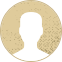Top Special Offer! Check discount

Get 13% off your first order - useTopStart13discount code now!

# the relationship between obese and blood pressure (bp) in a population

201 views 3 pages ~ 667 words
Get a Custom Essay Writer Just For You!

Experts in this subject field are ready to write an original essay following your instructions to the dot!

In order to establish the association between obesity and blood pressure (bp) in a population, an investigation with 102 participants was performed. The obese value denotes a numeric vector comprising the actual weight to ideal weight ratios. The bp value, on the other hand, represents a numeric vector containing systolic blood pressure. The male and female samples were drawn at random from the desired population. In this case, the obese number served as the independent variable, while the blood pressure data served as the dependent variable. The representation of quantitative data using graphs and pictorials is important in visual analysis especially for individuals who have less knowledge in statistics. This is because it is easy to draw conclusions from visual representation of data. For instance, in the pie chart above, it is evident that the number of female participants in the sample was high as compared to those of the male participants. From the set of data, the number of female participants was 58 which represent 57% and the male 44 representing 43% of the sample size. Apart from the pie chart, the bar graph below shows the similar results indicating that there were 14 female participants more than their counterparts.

Histogram

The histogram above resembles a normal distribution graph with two extreme outliers on the right hand side of the graph. Besides, there is a small difference between the mean, median and mode which means that most of the values are close to the mean. It should be noted that the graph above represents the systolic blood pressure irrespective of the sex.

Bp value

Descriptive Statistics

N

Range

Min

Max

Sum

Mean

Median

Mode

Std. Deviation

Variance

Bp

102

114

94

208

12,956

127.02

124

118

18.184

330.673

Valid N

102

The standard deviation in the bp set of values is low meaning that the most of the data points are closer to the mean. However, the high range value is attributed to the maximum outlier in the set of data.

Obese

Also, the histogram representing the obese values assumes the shape of a normal distribution graph. However, the data has three extreme values to the right hand side of the graph. The descriptive statistics obtained below show that the mode and median are close to the mean; implying that most of the data values are close to the mean. Besides, the obese values have a low standard deviation indicating that most of the data are closer to the mean.

Descriptive Statistics

N

Range

Min

Max

Sum

Mean

Median

Mode

Std. Deviation

Variance

Obese

102

1.58

0.81

2.39

133.93

1.3130

1.2850

1.2900

0.2578

0.066

Valid N

102

Correlation and Linear Regression

Correlation

The correlation analysis is a statistical tool that is used to measure the strength of the relationship between the independent and the dependent variable. For instance, the relationship between obese and blood pressure in our data set will be obtained through correlation analysis. There are several method used in determining the correlation of a given set of data. Some of these methods include the Spearman and the Pearson’s correlation. It should be noted that a correlation of -1 or +1 shows that the variables have a perfect relationship. However, a correlation of zero indicates that the variables exhibit no relationship.

The correlation coefficient between the obese and the bp data sets is 0.3261. This correlation is slightly above zero, implying that there is the presence of a weak positive relationship between being obese and having blood pressure. Also, a large increase in obese variable causes a small increase in the bp value.

Regression Statistics

The regression statistics table below indicates values of multiple R which represents the correlation between obese and bp values. Besides, the R squares value is obtained by squaring the correlation value. The column indicated coefficients give the constant terms of the linear regression line.

Regression Statistics

Multiple R

0.3261

R Square

0.1064

0.0974

Standard Error

17.2759

Observations

102

Coefficients

Intercept

96.8179

X Variable 1

23.0014

Therefore, the line of regression is given by,

Where, y represents the bp value,

X represents the obese values.

From the scatter plot graph, the data values are concentrated in one place accounting for the weak correlation coefficient of 0.3261.

May 24, 2023
Category:
Subcategory:
Subject area:
Number of pages

3

Number of words

667

53Rate:

4.7

Expertise Value
Verified writer

Clive2020 is an excellent writer who is an expert in Nursing and Healthcare. He has helped me earn the best grades with a theorists paper and the shadowing journal. Great job that always stands out!

Hire Writer

This sample could have been used by your fellow student... Get your own unique essay on any topic and submit it by the deadline.

Eliminate the stress of Research and Writing!

Hire one of our experts to create a completely original paper even in 3 hours!